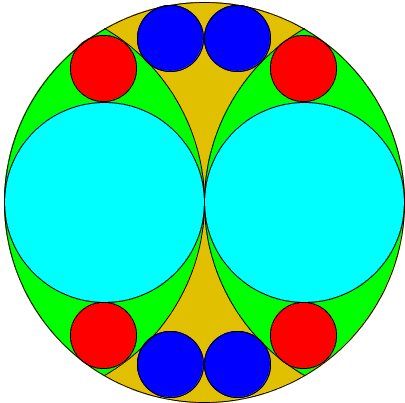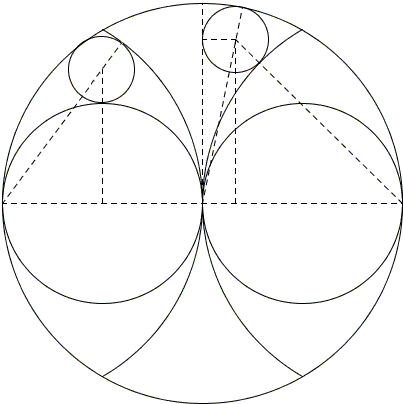# Peacock's Tail Sangaku

Many a sangaku deal with inscribed circles. While mostly elementary and solved easily by a few applications of Pythagorean theorem they were probably more a work of art than mathematics. Here's one such (to my taste) example. The sangaku was hung by a woman, Okuda Tsume, in the year 1865 at the Meiseirinji temple in Ogaki City, Gifu prefecture.In a circle of diameter 2R, draw two tangent arcs of radius R, and then ten inscribed circles, two of diameter R; four red of radius t and four blue of radius t'. Show that t = t' = R/6.

Solution

### References

1. H. Fukagawa, A. Rothman, Sacred Mathematics: Japanese Temple Geometry, Princeton University Press, 2008, p. 107In a circle of diameter 2R, draw two tangent arcs of radius R, and then ten inscribed circles, two of diameter R; four red of radius t and four blue of radius t'. Show that t = t' = R/6.

### Solution

The solution is based on the following diagramFor t we consider the left dashed triangle:

(R/2)² + (R/2 + t)² = (R - t)².

For t', consider the two right dashed triangles with a common altitude. Applying the Pythagorean theorem twice and equating the altitudes gives:

(R - t')² - (t')² = (R + t')² - (R - t')².

The two equations are easily manipulated into the desired identities.## Sangaku